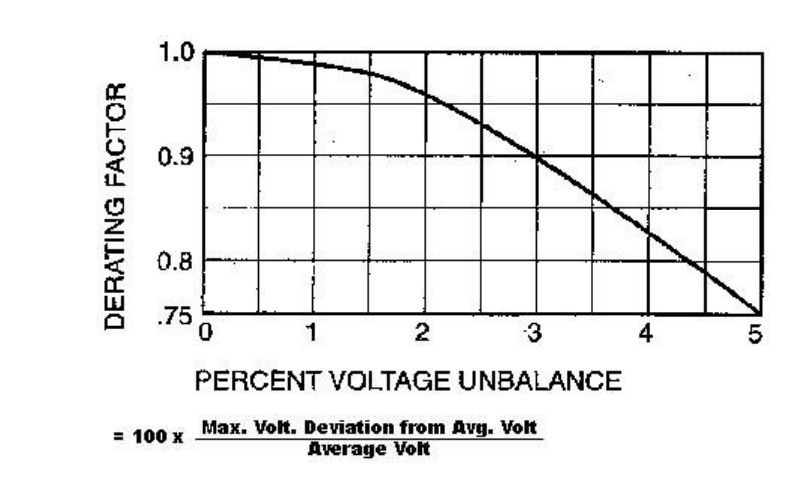# Voltage Imbalance

Effect of Voltage imbalance on Motor Performance

When the line voltages applied to a polyphase induction motor are not equal, imbalanced currents in the stator windings will result. A small percentage voltage imbalance will result in a much larger percentage current imbalance. Consequently, the temperature rise of the motor operating at a particular load and percentage voltage imbalance will be greater than for the motor operating under the same conditions with balanced voltages. Should voltages be imbalanced, the rated horsepower of the motor should be multiplied by the factor shown in the graph below to reduce the possibility of damage to the motor. Operation of the motor at above a 5 per cent voltage imbalance condition is not recommended. Alternating current, polyphase motors normally are designed to operate successfully under running conditions at rated load when the voltage imbalance at the motor terminals does not exceed 1 per cent. Performance will not necessarily be the same as when the motor is operating with a balanced voltage at the motor terminals.Example:
With voltage of 460, 467, and 450, the average is 459, the maximum deviation from the average is 9,
and the Per cent Imbalance = 100 x (9 / 459) = 1.96